# A Simple Mux Causes X-Pessimism in Gate Level Simulation

Make it to the Right and Larger Audience

Blog

# A Simple Mux Causes X-Pessimism in Gate Level Simulation

After RTL is synthesized, gate level simulation (GLS) is normally performed to check the correctness of netlist functionality. However, GLS is prone to a so-called x-pessimism issue where RTL and even real silicon can perform correctly and it is just in GLS ‘x’ is propagated and blocks normal function.

Here is a simple example. That “=1” gate is XOR gate. If Y is ‘x’, ‘x’ XOR ‘x’ is ‘x’ so Z is ‘x’ in GLS. Sounds reasonable but it is actually not the case. Since XOR two inputs are from the same source, ‘x’ XOR ‘x’ is either 0 XOR 0 or 1 XOR 0 and the resulted Z is always 0.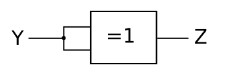Above example may not be realistic but it does illustrate the issue that when ‘x’ takes two paths and then converge, simulation tool does NOT take into consideration that ‘x’ in two paths are correlated and the end result may not be ‘x’.  This issue is called x-pessimism in GLS.

Below is more realistic example that a simple mux can cause x-pessimism in GLS. As a matter of fact this one is commonly seen across projects since mux is one of the basic building blocks.

A) is the mux design in RTL level. In netlist, it may be synthesized into AOI (and-or-invert) type of circuit in B) or into OAI (or-and-invert) type of circuit in C).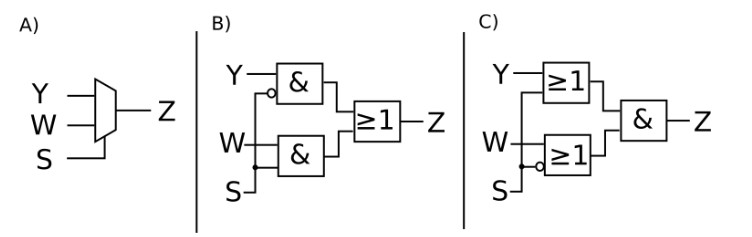A), B), C) are equivalent and their truth table are the same.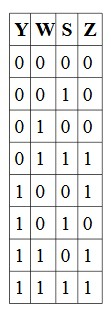But B) and C) can cause x-pessimism and A) does not. Let ‘s take a look of two cases

1. S is ‘x’ and Y=W=0
2. S is ‘x’ and Y=W=1

In case 1, Z is 0 in A) but Z is ‘x’ in C). In case 2, Z is 1 in A) but Z is ‘x’ in B). So only A) can generate correct Z value in both cases. It is important to note that B) and C) are correct circuit and there is NO issue on silicon. The ‘x’ is only GLS simulation issue.

Before we talk about how to resolve x-pessimism, we may ask why not instruct DC synthesizer to just use A) and not B)/C). B) and C) look more complicated and therefore slower and larger than a simple mux of A). Actually it is not the case. B) and C) have multiple gates and levels but they can be implemented efficiently in one-level CMOS gate. AOI and OAI are commonly used.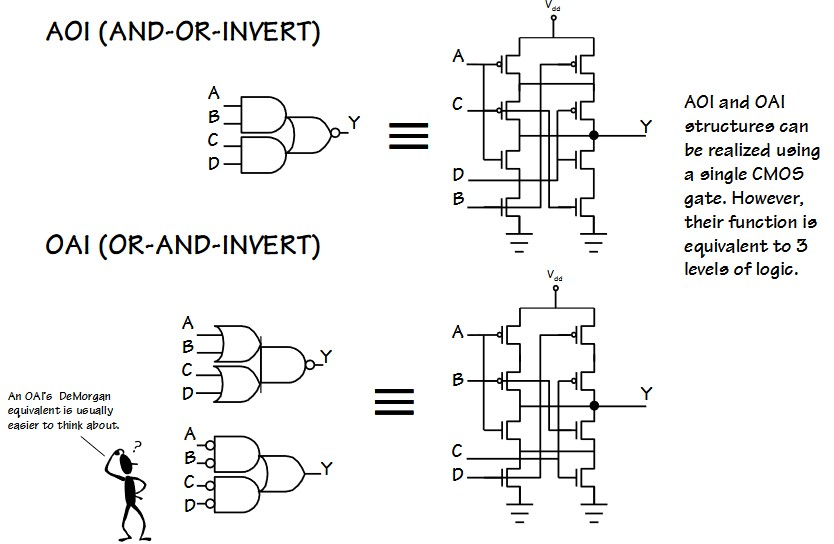To resolve mux x-pessimism, as mentioned above, we can ask synthesizer to keep mux type and not use AOI and OAI. We can also analyze GLS waveform, identify x-pessimism, and manually force GLS out of x. But this analysis is painful and easy to hide a real issue. These days there are commercial tools to do GLS x-pessimism recovery for you. A popular such tool is SimXACT from Avery System Design.

“SimXACT delivers an enhanced methodology for bringing up gate-level simulation more quickly and easily. SimXACT’s patented technology
can effectively analyze a simulation using a combinatorial analysis to determine if X values at D-inputs of DFF’s or ICG’s are real or false due
to X-pessimism. SimXACT automatically eliminates the false Xs by generating a set of HDL forces/releases which allows the gate-level
simulation to produce the correct results free of X-pessimism. The forces are applied on the fly during simulation to yield a one-pass
solution.”

Taking above mux issue as an example again, below diagram shows how SimXACT analyzes waveform and identifies where x-pessimism occurs.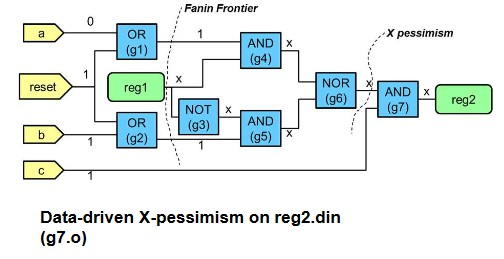Then SimXACT generates below force to get out of ‘x’. Note SimXACT runs with GLS and SimXACT does all this analysis and force on the fly. In other words, force takes effect with the simulation and it is not like dump result and apply force when rerun simulation.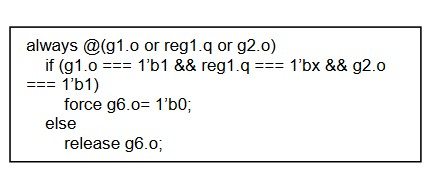Here is SimXACT flow.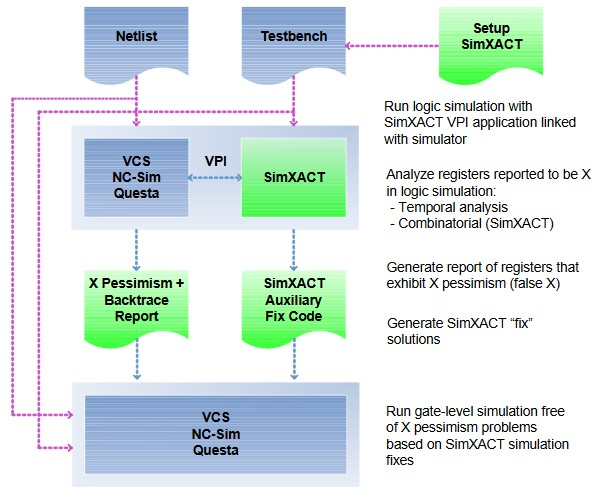For more details, you can refer to

Explanation of X pessimism effect in gate level simulation

SimXACT Accurate X VerificationAuthor brief is empty
Groups:

Tags: